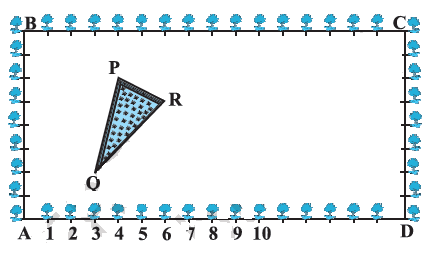# Ex.7.4 Q5 Coordinate Geometry Solution - NCERT Maths Class 10

Go back to  'Ex.7.4'

## Question

The class X students of a secondary school in Krishinagar have been allotted a rectangular plot of land for their gardening activity. Saplings of Gulmohar are planted on the boundary at a distance of $$1\; \rm m$$ from each other. There is a triangular grassy lawn in the plot as shown in the following figure. The students are to sow seeds of flowering plants on the remaining area of the plot.(i) Taking $$A$$ as origin, find the coordinates of the vertices of the triangle.

(ii) What will be the coordinates of the vertices of $$\Delta$$ $$PQR$$ if $$C$$ is the origin? Also calculate the areas of the triangles in these cases. What do you observe?

Video Solution
Coordinate Geometry
Ex 7.4 | Question 5

## Text Solution

What is known?

Saplings of Gulmohar are planted on the boundary at a distance of $$1 \rm\,m$$ from each other.

What is unknown?

• The coordinates of the vertices of the triangle.
• The coordinates of the vertices of $$\Delta$$ $$PQR$$ if $$C$$ is the origin.

Steps:

(i) Given,

Taking $$A$$ as origin, we will take $$AD$$ as $$x$$-axis and $$AB$$ as $$y$$- axis.

It can be observed from the figure that the coordinates of point $$P$$, $$Q$$, and $$R$$ are $$(4, 6)$$, $$(3, 2)$$, and $$(6, 5)$$ respectively.

Let,
\begin{align}\text{P}\left( {{x_1},{y_1}} \right) = \left( {4,6} \right)\end{align}
Let,
\begin{align}\text{Q} \left( {{x_2},{y_2}} \right) = \left( {3,{\text{ }}2} \right)\end{align}
Let,
\begin{align}\text{R}\,\,\left( {{x_3},{y_3}} \right) = \left( {6,5} \right)\end{align}

Area of a triangle

\begin{align}\!=\begin{bmatrix}\!\frac{1}{2}{x}_{1}\left({y}_{2}\!-\!{y}_{3}\right)\!+\!{x}_{2}\left({y}_{3}\!-\!{y}_{1}\right)\!+\!\\{x}_{3}\left({y}_{1}\!-\!{y}_{2}\right)\end{bmatrix} \end{align}\, \ldots(1)

By substituting the values of vertices $$P$$, $$Q$$, $$R$$ in the Equation (1),

Area of triangle

\begin{align} PQR &= \frac{1}{2}\,\,\left[ {{{{x}}_1}\left( {{{{y}}_2} - {{{y}}_3}} \right) + {{{x}}_2}\left( {{{{y}}_3} - {{{y}}_1}} \right) + {{{x}}_3}\left( {{{{y}}_1} - {{{y}}_2}} \right)} \right]\\&= \frac{1}{2}\,\,[4(2 - 5) + 3(5 - 6) + 6(6 - 2)]\\&= \frac{1}{2}\,[ - 12 - 3 + 24]\\&= \frac{9}{2}\,\;\rm{}Square\;units\end{align}

(ii) Given,

• Taking $$C$$ as origin, $$CB$$ as $$x$$-axis, and $$CD$$ as $$y$$-axis
• The coordinates of vertices $$P$$, $$Q$$, and $$R$$ are $$(12, 2)$$, $$(13, 6)$$, and $$(10, 3)$$ respectively.
• Let \begin{align}\text{P}\left( {x_1,y1} \right) = \left( {12, 2} \right)\end{align}
• Let \begin{align}{\text{Q}}\left( {x_2,y2} \right) = \left( {13, 6} \right)\end{align}
• Let \begin{align}\text{R}\left( {x_3,y3} \right) = \left( {10,3} \right)\end{align}

Area of a triangle

\begin{align}\!=\!\begin{bmatrix}\frac{1}{2} \;{x}_{1}\left({y}_{2}\!-\!{y}_{3}\right)\!+\!{x}_{2}\left({y}_{3}\!-\!{y}_{1}\right)\!+\!\\{x}_{3}\left({y}_{1}\!-\!{y}_{2}\right)\end{bmatrix} \end{align} \ldots (1)

By substituting the values of vertices $$P$$, $$Q$$, $$R$$ in the Equation ($$1$$),

Area of triangle

\begin{align} PQR & \!=\! \frac{1}{2}\begin{bmatrix} {x_1}( {{y_2} \!-\! {y_3}} ) \!+\! {x_2}( {{y_3} \!-\! {y_1}} ) \!+\!\\ {x_3}( {{y_1} \!-\! {y_2} )} \end{bmatrix} \\&\!=\! \frac{1}{2}\begin{bmatrix} 12(6 \!- \!3) \!+\! 13(3 \!-\! 2)\!+\! \\ 10(2\! - 6) \end{bmatrix} \\ &\!= \!\frac{1}{2}\left[ {36 \!-\! 13 \!+\! 40} \right]\\ &\!=\! \frac{9}{2}\;\rm{}Square \;units \end{align}

It can be observed that the area of the triangle is same in both the cases.

Learn from the best math teachers and top your exams

• Live one on one classroom and doubt clearing
• Practice worksheets in and after class for conceptual clarity
• Personalized curriculum to keep up with school